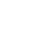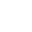# your analysis and valuation process

### Analystix Tools

A package of 5 Excel Add-ins that help you manipulate and analyze data, and simplify some of the calculations performed during the analysis and valuation process.

### Knowledge Base

Financial tutorials & resources to assist in financial analysis and valuation, including forecasting, cost of capital estimation, useful links and more.

### Monthly Market Update

Monthly update of various market parameters, including pricing and leverage ratios, risk free yields and corporate spreads, and GDP growth forecasts.

### Analystix Tools

##### Histogram Builder

Analyze the distribution of your data by creating histograms

##### Data Arranger

Rearrange periodic data, fill empty data and calculate averages and end of period

##### Cost of Capital Calculator

Easily calculate Cost of Equity and WACC. Includes Beta, Size Premium and Debt Margin calculators

##### CAGR Calculator

Calculate growth compared to previous and parallel periods or average annual growth rate and CAGR

##### B&S Calculator

Calculate the call and put values of an option using the Black and Scholes formula

Since most of us use Excel as our main financial analysis and valuation tool, we think Excel Add-ins that automate some of the workflow is the best way to improve and simplify our work.### Financial Resources & Tutorials

The Financial tutorials & resources section is a knowledge base of articles, tutorials and resources that can assist you in financial analysis and valuation:
• Modeling and forecasting tutorials
• Specific industry forecasting ideas
• Cost of Capital calculation tutorials and resources

### Monthly Market Update

The Analystix Monthly Market Update provides updated market data:
• Stock Indices Returns
• Pricing Multiples Distribution
• Leverage Ratios Distributions
• Yield Curves